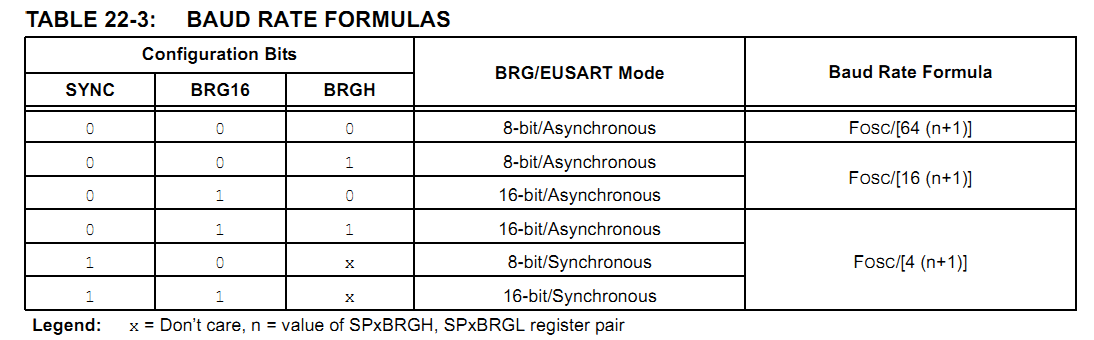# I have doubt in PIC16f1527 baud rate formula

Status
Not open for further replies.

#### ragav4456

##### Full Member level 4
Hi friends

I have doubt in UART baudrate formula

SYNC=BRGH=BRG16=0, formula FOSC/[64(n+1)]
SYNC=BRG16=0,BRGH=1, formula FOSC/[16(n+1)]

in my program SYNC=0;BRG16=1;BRGH=1;

can i use this formula
SYNC=0;BRGH=BRG16=1, formula FOSC/[4(n+1)]

Yes, in fact that is the formula you have to use. Just refer to the table in the datasheet:Hope this helps.
Tahmid.

kindly check the program

How to change the formula?
Code:
#include <htc.h>
#include "sci.h"

unsigned int uiRS232Error = 0;

unsigned char sci_Init(unsigned long int baud, unsigned char ninebits)
{
int X;
unsigned long tmp;
uiRS232Error = 0;

tmp = 16UL * baud;
X = (int)(FOSC/tmp) - 1;
if((X>255) || (X<0))
{
tmp = 64UL * baud;
X = (int)(FOSC/tmp) - 1;
if((X>255) || (X<0))
{
return 1;
}
else

TX1STAbits.BRGH = 0;	/* low baud rate */
}
else
TX1STAbits.BRGH = 1;	/* high baud rate */
SPBRG = X;	/* set the baud rate */
BAUD1CONbits.BRG16=1;
BAUD1CONbits.ABDOVF=0;
BAUD1CONbits.RCIDL=0;
BAUD1CONbits.SCKP=0;
BAUD1CONbits.WUE=0;
BAUD1CONbits.ABDEN=1;
TX1STAbits.SYNC = 0;           /* asynchronous */
TX1STAbits.SENDB = 0;

RC1STAbits.SPEN = 1;	/* enable serial port pins */
RC1STAbits.CREN = 1;	/* enable reception */
RC1STAbits.SREN = 0;	/* no effect */
PIE1bits.TX1IE = 1;	/* disable tx interrupts */
PIE1bits.RC1IE = 1;	/* disable rx interrupts */
TX1STAbits.TX9  = ninebits?1:0;	/* 8- or 9-bit transmission */
RC1STAbits.RX9  = ninebits?1:0;	/* 8- or 9-bit reception */
TX1STAbits.TXEN = 1;	/* enable the transmitter */
PIR1bits.TX1IF = 0;	/* enable tx interrupts */
PIR1bits.RC1IF = 0; /* enable rx flag */

return 0;
}

void sci_PutByte(unsigned char byte)
{	unsigned long ulICnt=0;

uiRS232Error = 0;

while(!PIR1bits.TX1IF && ulICnt < WAIT_TICK_MAX)
{	ulICnt++;
}
TX1REG = byte;

if(ulICnt >= WAIT_TICK_MAX)
{	uiRS232Error = 1;
}
return;
}

unsigned char sci_GetByte(void)
{	unsigned long ulICnt=0;

uiRS232Error = 0;

while(!PIR1bits.RC1IF && ulICnt < WAIT_TICK_MAX)
{	ulICnt++;
}

sci_CheckOERR();

if(ulICnt >= WAIT_TICK_MAX)
{	uiRS232Error = 1;
}

return RC1REG;	/* RXD9 and FERR are gone now */
}

unsigned char sci_CheckOERR(void)
{
if(RC1STAbits.OERR)
{
RC1STAbits.CREN = 0;
RC1STAbits.CREN = 1;
return 1;
}

return 0;
}

#define sci_PutNinth(bitnine)	(TX9D = bitnine?1:0;)

unsigned char sci_GetNinth(void)
{
while(!PIR1bits.RC1IF)
continue;

return RC1STAbits.RX9D;
}

unsigned char sci_GetFERR(void)
{
while(!PIR1bits.RC1IF)
continue;

return RC1STAbits.FERR;
}

Last edited:

Status
Not open for further replies.